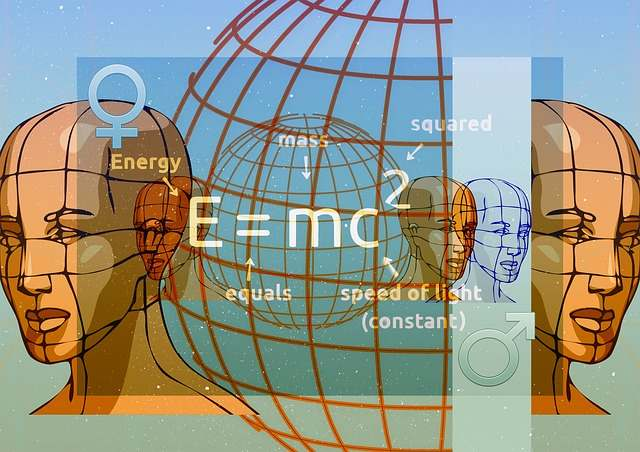# Finite Math Help

## Why is Finite Math so Hard for Students?

 We offer you quality and prompt Math Homework Help Online. Our service is convenient, efficient and confidential. We can solve Math problems for you. Our rate starts \$35/hour. We provide a free quote in hours. Quick turnaround!## Finite Math Formulas

$x = \displaystyle \frac{-b \pm \sqrt{b^2 - 4ac}}{2a}$ $\displaystyle \Pr\left( a \le X\le b \right) = \Pr\left( \frac{a-\mu}{\sigma} \le Z \le \frac{b-\mu}{\sigma} \right)$